# Layout Algorithms

This topic describes the layout algorithms used by the TreeMap control, and consists of the following sections.

## Overview

The TreeMap control allows you to specify the algorithm used to layout tree map items. The Squarified algorithm is used by default.

To change the algorithm used to arrange tree map items, assign the required ITreeMapLayoutAlgorithm class descendant object to the TreeMapControl.LayoutAlgorithm property.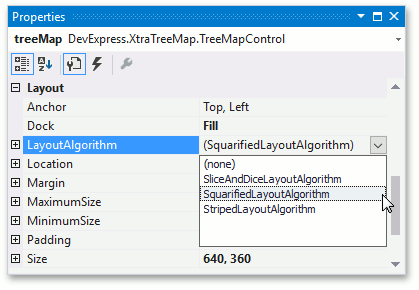After that, customize algorithm settings. For example, all predefined algorithms support fill direction customization. To do this, use the TreeMapLayoutAlgorithmBase.Direction property.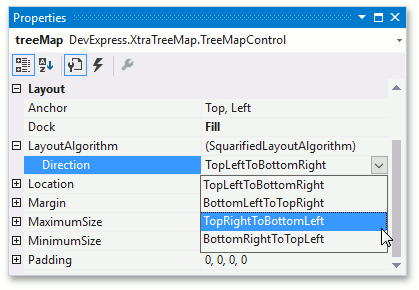The following sections describe predefined algorithm behavior.

## Slice and Dice Layout Algorithm

This layout algorithm divides the space between items, slicing it in the specified direction depending on item value.

The following image demonstrates the country GDP tree map with a layout calculated using this algorithm.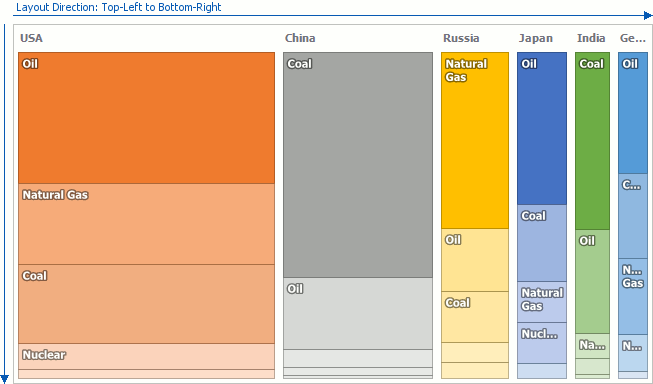## Squarified Layout Algorithm

The Squarified algorithm lays out items, so that the width/height ratio will be closer to 1. In other words, this algorithm tries to square the layout.

The following image demonstrates the tree map layout calculated using this algorithm.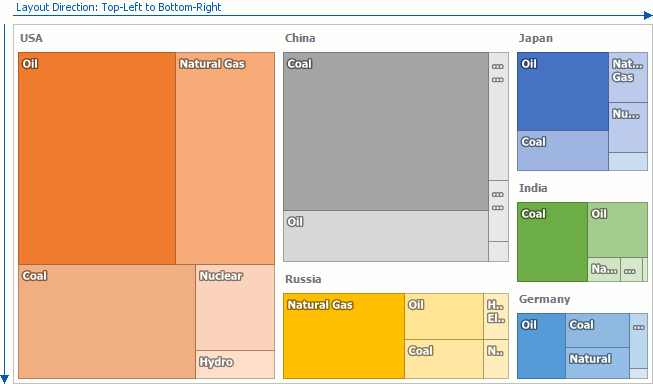## Striped Layout Algorithm

This algorithm is a modified version of the Squarified algorithm. The main distinction of the Striped algorithm consists in preserving tree map item orientation, e.g., item width, calculated using this algorithm, is larger than height.

The following image demonstrates the layout calculated using this algorithm.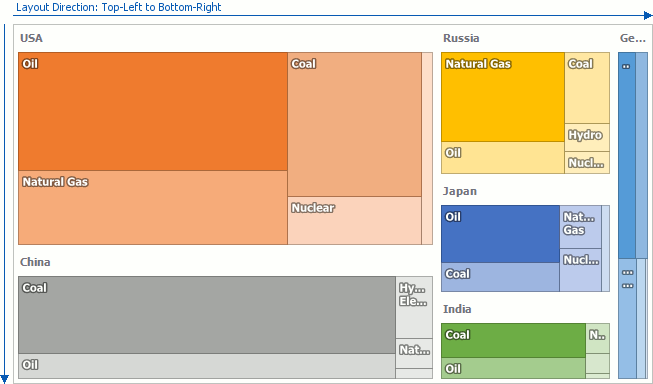## Custom Layout Algorithm

In addition to the predefined layout algorithms, it is possible to implement a custom layout algorithm. To do this, design a class derived from the ITreeMapLayoutAlgorithm and implement the ITreeMapLayoutAlgorithm.Calculate method.

Refer to the How to: Implement a Custom Layout Algorithm example to learn more.